# Linear estimator

(diff) ← Older revision | Latest revision (diff) | Newer revision → (diff)

A linear function of observable random variables, used (when the actual values of the observed variables are substituted into it) as an approximate value (estimate) of an unknown parameter of the stochastic model under analysis (see Statistical estimator). The special selection of the class of linear estimators is justified for the following reasons. Linear estimators lend themselves more easily to statistical analysis, in particular to the investigation of consistency, unbiasedness, efficiency, the construction of corresponding confidence intervals, etc. At the same time, in a fairly wide range of cases the search for "optimal" (in a well-defined sense) estimators does not lead beyond the limits of the class of linear estimators. For example, the statistical analysis of a linear regression model (see Linear regression) of the formgives as best linear unbiased estimator of the parameterthe least-squares estimator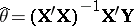(linear with respect to the observed values of the random variableunder investigation). Hereis the-dimensional column vector of observed values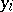,, of the resulting test (random variable) under investigation,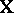is the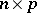matrix (of rank) of observed values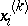,,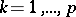, ofnon-random factor arguments on which the resulting testdepends,is the-dimensional column vector of the unknown parameters,, andis the-dimensional random column vector of residual components, which satisfies the condition,(being the unit matrix).

How to Cite This Entry:
Linear estimator. Encyclopedia of Mathematics. URL: http://encyclopediaofmath.org/index.php?title=Linear_estimator&oldid=11992
This article was adapted from an original article by S.A. Aivazyan (originator), which appeared in Encyclopedia of Mathematics - ISBN 1402006098. See original article